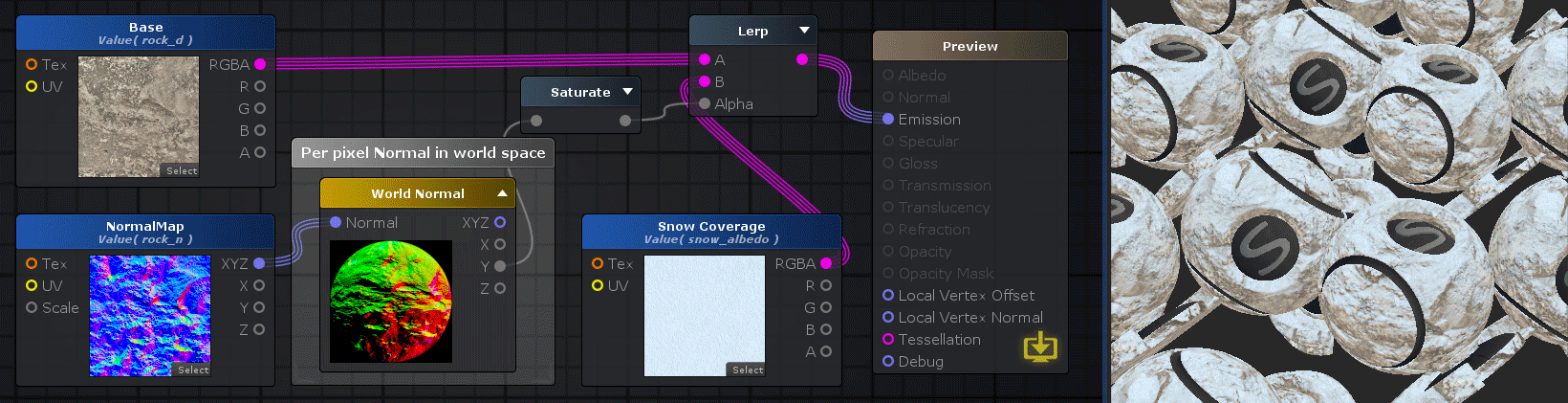# Unity Products:Amplify Shader Editor/World Normal

Jump to: navigation, search

## World Normal Node

Per pixel calculation of a vector that represents the surface normal in world space. A normal vector is a unit vector perpendicular to the surface that points to where surface is faced. This data is very useful and common for lighting calculation. This node also allows to receive a normal vector in tangent space to shift the normals around. The most common use case would be to use a normal map texture in tangent space to perturb the object's surface normals.

NOTE: Do not confuse with Vertex Normal node.Nodes used: Texture Sample, World Normal, Saturate, Lerp

Node Parameter Description Default Value
Normalize If toggled on, forces a Normalize operation over the world normal vector. Off
Input Port Description Type
Normal Normal vector in tangent space, if left unconnected uses a default constant tangent vector3 of ( 0, 0, 1 ). Float3
Output Port Description Type
XYZ Returns the surface normal vector in world space. Float3
X Returns only the X component of the world normal vector. Float
Y Returns only the Y component of the world normal vector. Float
Z Returns only the Z component of the world normal vector. Float